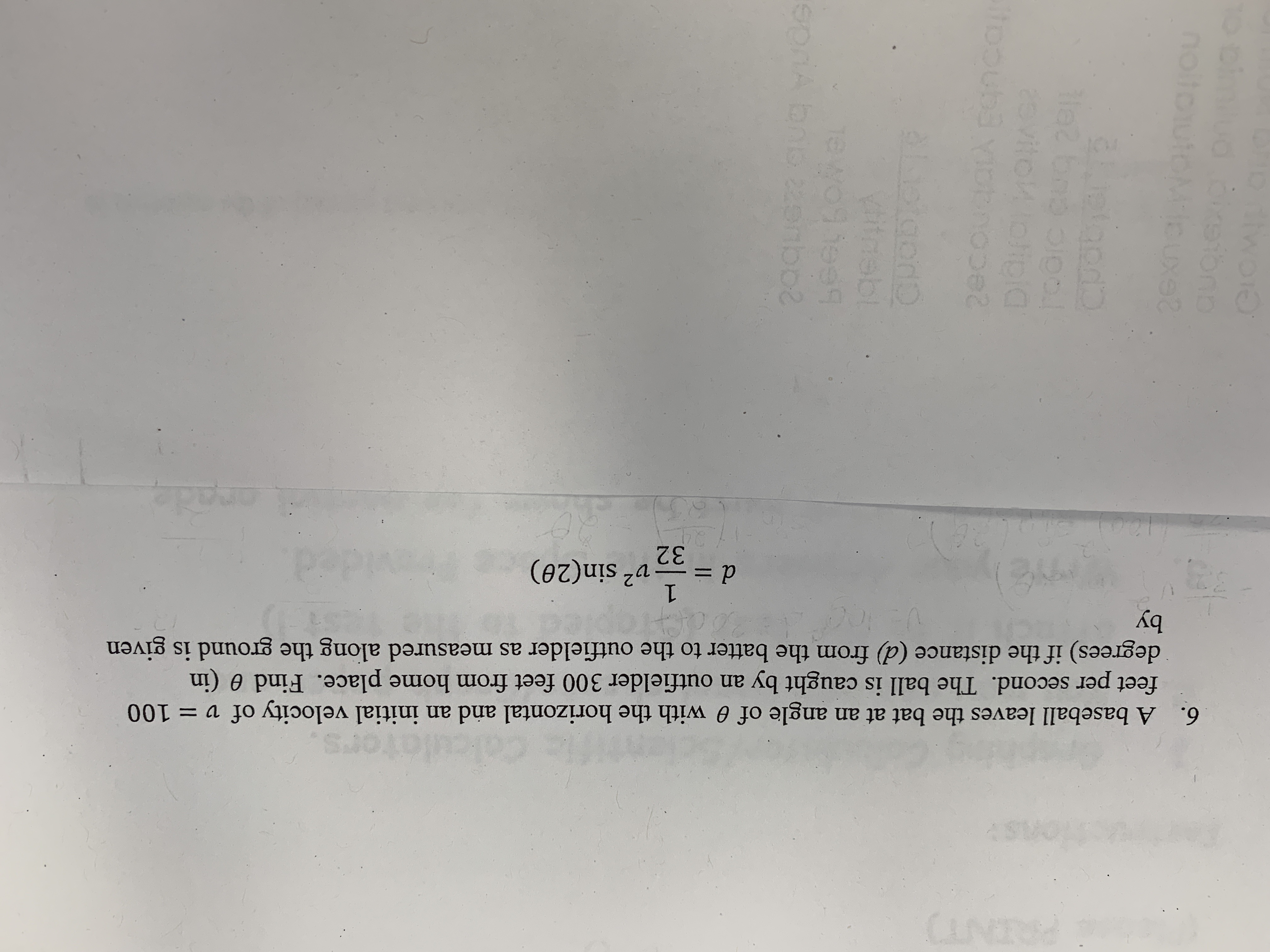# A baseball leaves the bat at an angle offeet per second. The ball is caught by an outfielder 300 feet from home place. Find 0 (indegrees) if the distance (d) from the batter to the outfielder as measured along the ground is givenby0 with the horizontal and an initial velocity of v 1006.d n(20)32

Question
6 views

A baseball leaves the bat an an angle of theta with the horizontal and an inital velocity of v = 100 feet per second. The ball is caught by an outfielder 300 feet from home place. Find theta (in degrees) if the distance (d) from the batter to the outfieldre as measures along the ground is given by d=(1/32)(v2)sin(2theta)help_outlineImage TranscriptioncloseA baseball leaves the bat at an angle of feet per second. The ball is caught by an outfielder 300 feet from home place. Find 0 (in degrees) if the distance (d) from the batter to the outfielder as measured along the ground is given by 0 with the horizontal and an initial velocity of v 100 6. d n(20) 32 fullscreen
check_circle

Step 1

According to the question, a baseball leaves the bat an an angle of theta with the horizontal and an inital velocity of v = 100 feet per second.

The ball is caught by an outfielder 300 feet from the home place.

Step 2

According to the given condition, the relation between the velocity (v)  and the diatance (d) can be expressed as:

Step 3

Now, in this case, velocity (v)=100 feet per sec and distance (d)=300 feet.

Therefore,...

### Want to see the full answer?

See Solution

#### Want to see this answer and more?

Solutions are written by subject experts who are available 24/7. Questions are typically answered within 1 hour.*

See Solution
*Response times may vary by subject and question.
Tagged in

### Inverse Trigonometric Ratios